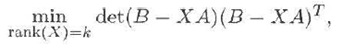# Algorithms in data mining : reduced rank regression and classification by tensor methods

Abstract: In many fields of science, engineering, and economics large amounts of data are stored and there is a need to analyze these data in order to extract information for various purposes. Data mining is a general concept involving different tools for performing this kind of analysis. The development of mathematical models and efficient algorithms is of key importance. In this thesis, which consists of three appended manuscripts, we discuss algorithms for reduced rank regression and for classification in the context of tensor theory.The first two manuscripts deal with the reduced rank regression problem, which is encountered in the field of state-space subspace system identification. More specifically the problem iswhere A and B are given matrices and we want to find X under a certain rank condition that minimizes the determinant. This problem is not properly stated since it involves implicit assumptions on A and B so that (B - XA)(B - XA)T is never singular. This deficiency of the determinant criterion is fixed by generalizing the minimization criterion to rank reduction and volume minimization of the objective matrix. The volume of a matrix is defined as the product of its nonzero singular values. We give an algorithm that solves the generalized problem and identify properties of the input and output signals causing singularity on the objective matrix.Classification problems occur in many applications. The task is to determine the label or class of an unknown object. The third appended manuscript concerns with classification of hand written digits in the context of tensors or multidimensional data arrays. Tensor theory is also an area that attracts more and more attention because of the multidimensional structure of the collected data in a various applications. Two classification algorithms are given based on the higher order singular value decomposition (HOSVD). The main algorithm makes a data reduction using HOSVD of 98%- 99% prior the construction of the class models. The models are computed as a set of orthonormal bases spanning the dominant subspaces for the different classes. An unknown digit is expressed as a linear combination of the basis vectors. The amount of computations is fairly low and the performance reasonably good, 5% in error rate.This dissertation MIGHT be available in PDF-format. Check this page to see if it is available for download.